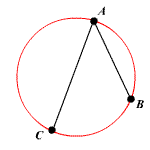# Chord

A chord of a circle is a line segment with both endpoints on the boundary.In the figure, $\stackrel{¯}{AB}$ and $\stackrel{¯}{AC}$ are chords of the circle.

A diameter is a chord which passes through the center of the circle and is the longest possible length for that circle.

Some basic theorems involving chords:

• In the same circle or in congruent circles, two minor arcs are congruent if and only if their corresponding chords are congruent.

• In the same circle or in congruent circles, two chords are congruent if and only if they are equidistant from the center.